##CS 660: Combinatorial Algorithms Splay Tree

[To Lecture Notes Index]
San Diego State University -- This page last updated Sept 27, 1995## Self-Organizing BST

Basic Rotation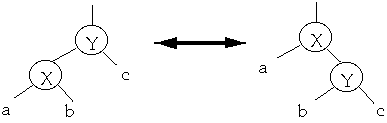Simple Exchange (Transpose)
When we access a node, apply a rotation to move the node one level closer to root
If each node is accessed with probability of 1/n the average search time is: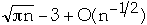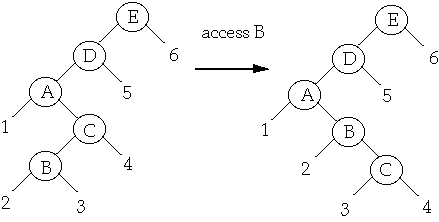Move-to-root
When we access a node, apply series of rotations to make the node the root
We have a list of n items: a1, a2, ..., an
Probability of accessing item ak is P(ak)
The average search cost is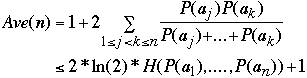Where H(P(a1), ....P(an)) is the entropy of the distribution
If P(ak) = 1/n thenMove-to-root example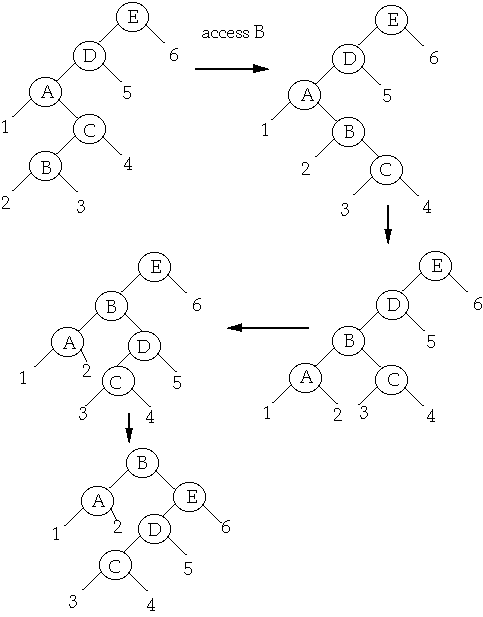### Splay Trees

Splaying

Splay step at x

let p(x) = parent of node x

case 1 (zig) p(x) = root of the treecase 2 (zig-zig) p(x) is not the root and x and p(x) are both left (right) children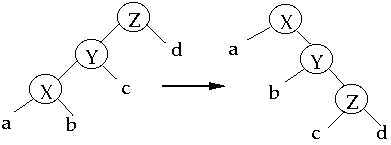case 3 (zig-zag) p(x) is not the root and x is a left (right) child and p(x) is a right(left ) child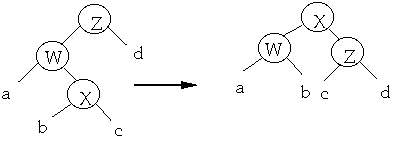To Splay a node X, repeat the splay step on X until it is the root
Splay B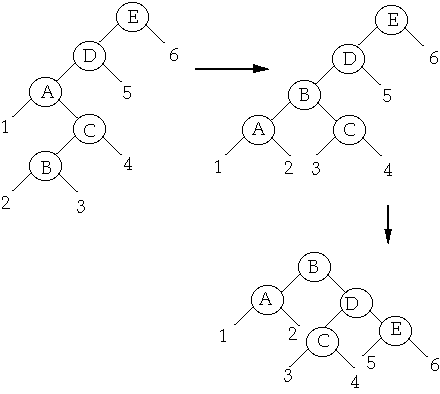Splay vs. Move-to-root

Case 1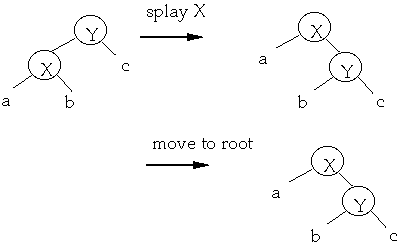Case 2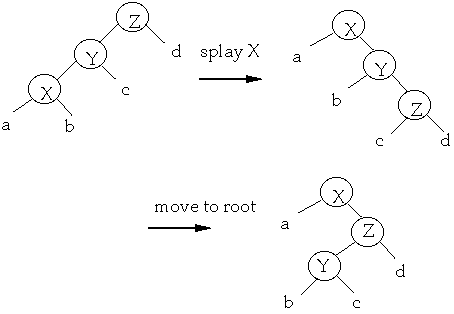Splay vs. Move-to-root

Case 3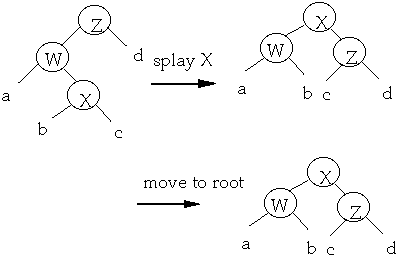Move-to-root A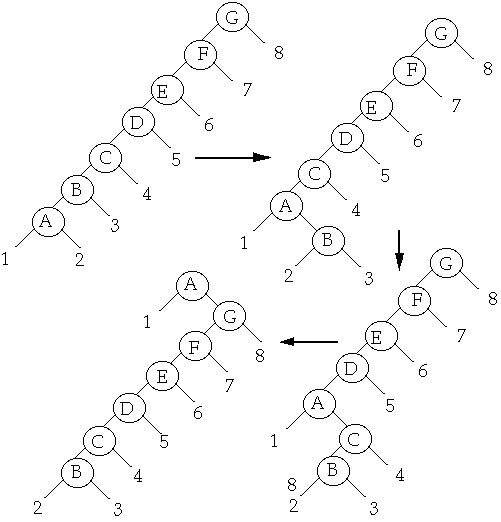Splay A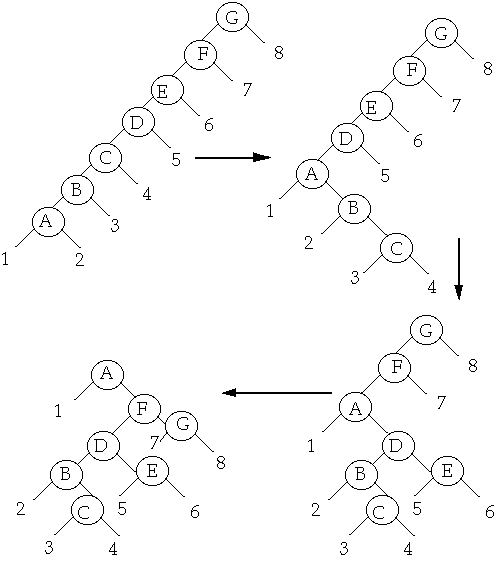#### Performance of Splay Tree

Splaying at a node of depth d takes Theta(d) time

ck= actual cost of operation k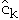= amortized cost of operation k

Dk = the state of the data structure after applying k'th operation to Dk= potential associated with Dk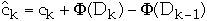so we get: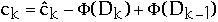The actual amount of work required is given by: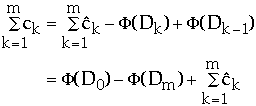So need the total amortized work and difference in potential
Potential for Splay Trees

Let:
w(x) = weight of node x, a fixed but arbitrary value
size(x) =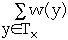rank(x) = lg(size(x))Example

Let w(x) = 1/n where n is the number of nodes in the treeLemma The amortized time to splay node x in a tree with root at t is at most 3(r(t) - r(x)) + 1 = O(lg(s(t)/s(x)))
Let s, r denote the size, rank functions before a splay
Let s', r' denote the size, rank functions after a splay
Count rotations

Case 1 (zig) One rotation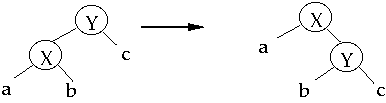Amortized time of this step is:1 + [r'(x) + r'(y)] - r(x) - r(y) only x and y change rank
<= 1 + r'(x) - r(x)
r(y) >= r'(y)
<= 1 + 3(r'(x) - r(x))
r'(x) >= r(x)

Case 2 (zig-zig) Two rotationsAmortized time of this step is:

2 + r'(x) + r'(y) + r'(z)
- r(x) - r(y) - r(z) only x, y ,z change rank
= 2 + r'(y) + r'(z) - r(x) - r(y)
r'(x) = r(z)
<= 2 + r'(x) + r'(z) - 2r(x)
r'(x) >= r'(y) and
r(y) >= r(x)

Assume that 2r'(x) - r(x) - r'(z) >= 2

2 + r'(x) + r'(z) - 2r(x)
<= 2r'(x) - r(x) - r'(z) + r'(x) + r'(z) - 2r(x)
= 3r'(x) - 3r(x)
Need to show 2r'(x) - r(x) - r'(z) >= 2

Claim 1Set b = 1-a
We have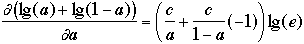Setting this to 0 to find extreme value we get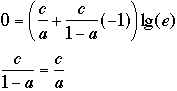so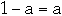that is a = 1/2 and b = 1/2
but lg(1/2)+lg(1/2)= -2

End claim 1

Claim 2 2r'(x) - r(x) - r'(z) >= 2
Recall that:We have:
r(x) + r'(z) - 2r'(x) = lg(s(x)) + lg(s'(z)) - 2lg(s'(x))
= lg(s(x)/s'(x)) + lg(s'(z)/s'(x))
Now s(x) + s'(z) <= s'(x)
(Why?)
so
0<= s(x)/s'(x) + s'(z)/s'(x) <= 1
Set s(x)/s'(x) = a and s'(z)/s'(x) =b in claim 1 to get
lg(s(x)/s'(x)) + lg(s'(z)/s'(x)) <= -2
Thus r(x) + r'(z) - 2r'(x) <= -2 or 2r'(x) - r(x) - r'(z) >= 2Case 3 (zig-zag)Amortized time of this step is:

2 + r'(x) + r'(w) + r'(z)
- r(x) - r(w) - r(z)
<= 2 + r'(w) + r'(z) - 2r(x)
r'(x) = r(z) and
r(w) >= r(x)

Assume that 2r'(x) - r'(w) - r'(z) >= 2

2 + r'(w) + r'(z) - 2r(x) <= [2r'(x) - r'(w) - r'(z)] + r'(w) + r'(z) - 2r(x)

= 2r'(x) - 2r(x) <= 3 * ( r'(x) - r(x) )

Claim 3: 2r'(x) - r'(w) - r'(z) >= 2
Proof: see claims 1 & 2

Putting it All together

Lemma The amortized time to splay node x in a tree with root at t is at most 3(r(t) - r(x)) + 1 = O(lg(s(t)/s(x)))
Splay at B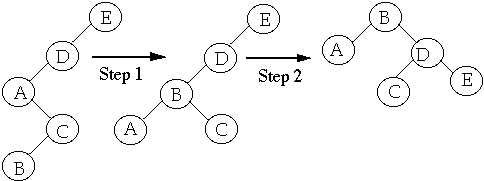Cost of Step 1 <= 3* ( r'(B) - r(B) ) case 3

Cost of Step 2 <= 3 * ( r''(B) - r'(B) ) case 2

Total cost = 3* ( r'(B) - r(B) ) + 3 * ( r''(B) - r'(B) )
= 3 * ( r''(B) - r(B) )
= 3 * ( r(E) - r(B) )

Case 1 only happens when splaying a child of the root

This happens at most once per splay
Amortized Cost of M Splay Operations
on Tree with N nodes

Let node i be accessed q(i) times.

Then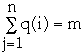Theorem (Balance Theorem) The total access time is
O( (m + n) * lg (n + m) )

Theorem (Static Optimality) If every item is accessed at least once, then the total access time is: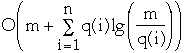Example

Let q( i ) = 1 then we haveproof of Static Optimality:

Recall that:
size(x) =rank(x) = lg(size(x))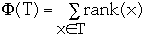let w(i) = q(i)/m then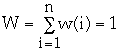We wish to compute the actual cost of m operations.

Recall:So we need to compute the change in potential and the amortized cost over m operations.
First the change in potential.

The biggest change in potential comes when a node moves from root to a leaf.

Assume that all nodes start at the root and end up as a leaf.

This will give us an upper bound on the change in potential.

We have:
rank of the root = lg(W)
rank of node i as a leaf = lg( w( i ) )

So change in rank of node i is at most
lg(W) - lg( w( i ) ) = lg( W/w(i) )

so the upper bound on the net decrease in potential over m operations is:The amortized access time of item i is: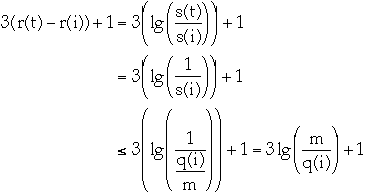Amortized access of all times is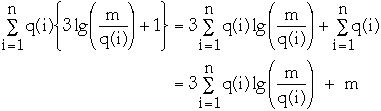So the total cost is bounded by: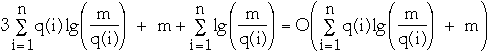#### Splay Operations

access(i, t): if i is in tree t return pointer to i, otherwise return null pointer
Find i, then splay tree t at i.
If i is not in tree t, then splay last node accessed looking for i

join (a, b): Return tree formed by combining tree "a", and tree "b". Assumes that every item in "a" has key less then every item in "b"
Splay largest item in "a", then add "b" as a right child of root of "a"

split (i, t): Split tree t, containing item i, into two trees: "a", containing all items with key less or equal to "i"; and "b", containing all items with key greater than "i"
Perform access(i, t) then split tree at root

insert(i, t): insert i in tree t
Perform split (i, t) then make i the root of the two trees returned by split

delete(i, t): delete i from tree t
Perform access(i, t) then perform join on t's subtrees

Balance Theorem with Updates

A sequence of m arbitrary operations on a collection of initially empty splay trees takes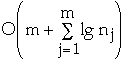time, where nj is the number of items in the tree or trees involved in operation j.

#### Top-Down Splay

Case 1 X is the node we are splaying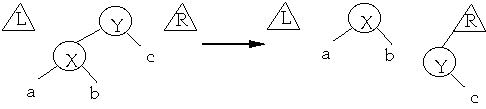Case 2 (zig-zig) The node we are splaying is in the subtree rooted at X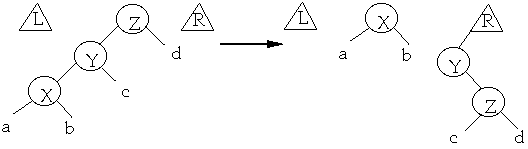Case 3 (zig-zag) The node we are splaying is in the subtree rooted at X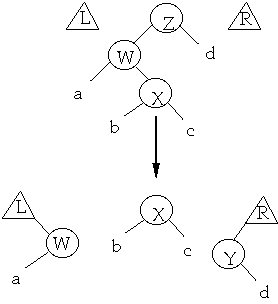Case 4 - The last step: X is the node we wish to splay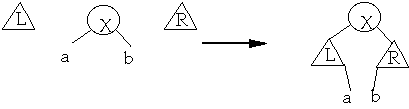Example Splay at B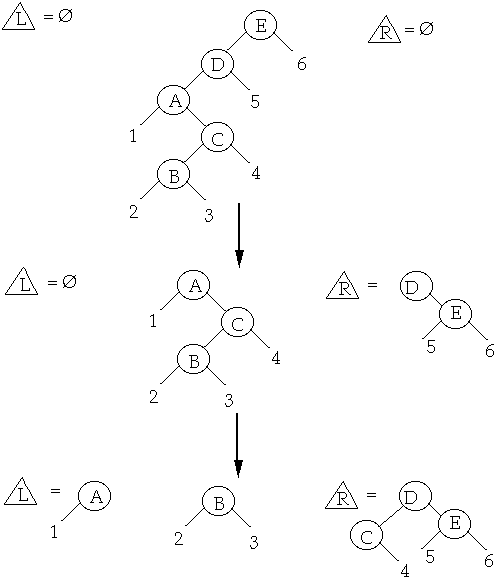Example Splay at B